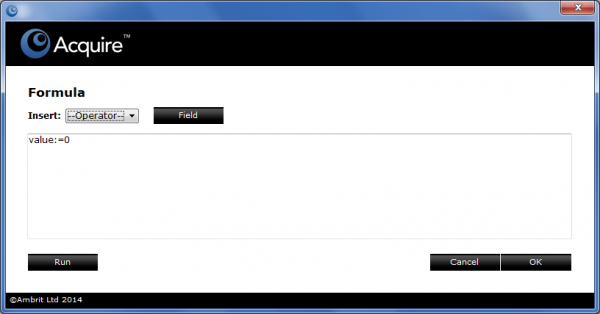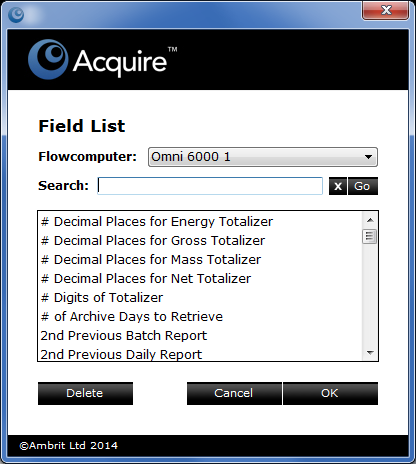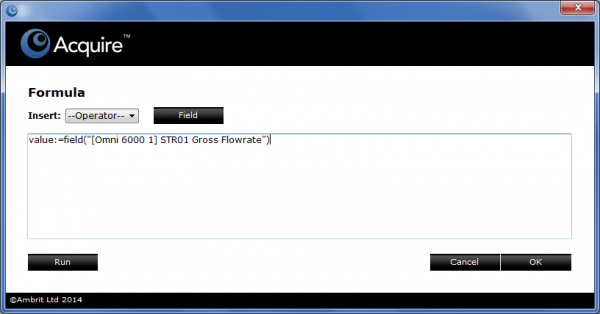# Adding a Formula to a Field

This is an optional feature and will only be available if it has been licensed in the key file.

Acquire must be in Config mode to perform these steps.

The formula editor allows a simple single-line formula to be added to a calculation field or flow computer field. Formulas can also be used to aggregate fields from multiple flow computers.

## 1. Click the ‘Edit’ button adjacent to the formula field

The Fomula window will be displayed:## 2. Enter the formula

Two types of formulas can be defined: read formulas and write formulas.

Read formulas take values from the flow computers and perform the calculation for display purposes.

Read formulas have the syntax value:=expression where expression is a mathematical formula e.g. value:=value*1000.

Write formulas

Write formulas take values from the flow computers, perform the calculation and then write the result back to the field in the flow computer. Write formulas can only be applied to flow computer and RTU fields.

Write formulas have the syntax writevalue:=expression where expression is a mathematical formula e.g. writevalue:=writevalue/1000.

Scripting

Any field from any of the flowcomputers can be selected and can be included in the calculation.

eg value:=field(“[Omni]STR01 Pressure”)

Multiple fields can also be included in the calculation:

eg value:=field(“[Omni]STR01 Gross Flowrate”)+field([Omni]STR02 Gross Flowrate”)

These fields can also come from multiple flowcomputers:

eg value:=field(“[Omni 1]STN01 Gross Total”)+field([Omni 2]STN01 Gross Total”)

Variables can be declared and used as part of the calculation:

• var a := 2;
• var b := 3;
• value := a + b;

If statements can also be written to display a value based on a Boolean expression being satisfied. The following formula returns the difference between the limit:

```var temperature := field("[Omni 6000]Temperature");
var high_temperature := field("[Omni 6000]Temp Hi-Lim");
if (temperature > high_temperature)
value := temperature - high_temperature;
else
var low_temperature := field("[Omni 6000]Temp Lo-Lim");
if (temperature < low_temperature)
value := temperature - low_temperature;
else
value := 0;
end if
end if```

Operators

The calculation can include any of the following operators. A full list is available in the operator popup menu.

 + plus - minus * multiply / divide % mod \ integer divide = equals # not equal to > greater than < less than >= greater than or equal to <= less than or equal to || Logical OR & Logical AND | Bitwise OR && Bitwise AND << Bit Rotate Left >> Bit Rotate Right ? Bit Test ! not abs absolute value, i.e. always positive

## 3. Click the ‘Field’ button

The Field List window for the selected flow computer will be displayed:• Select the flow computer from the ‘Flowcomputer’ popup menu
• Enter the field name (or part of) in the ‘Search’ field and click the ’Go’ button
• Click on the field in the field list to highlight it

## 4. Click the ‘OK’ button

The selected field will be added to the formula:## 5. Click the ‘Run’ button

This will test that the formula’s syntax and the returned value are correct:## 6. Click the ‘OK’ button

The Formula window will be displayed again:## 6. Click the ‘OK’ button

The formula will be saved.